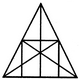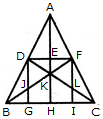# Non Verbal Reasoning - Analytical Reasoning - Discussion

Discussion Forum : Analytical Reasoning - Section 1 (Q.No. 25)
25.

What is the number of straight lines and the number of triangles in the given figure.10 straight lines and 34 triangles
9 straight lines and 34 triangles
9 straight lines and 36 triangles
10 straight lines and 36 triangles
Explanation:

The figure may be labelled as shown.The Horizontal lines are DF and BC i.e. 2 in number.

The Vertical lines are DG, AH and FI i.e. 3 in number.

The Slanting lines are AB, AC, BF and DC i.e. 4 in number.

Thus, there are 2 + 3 + 4 = 9 straight lines in the figure.

Now, we shall count the number of triangles in the figure.

The simplest triangles are ADE, AEF, DEK, EFK, DJK, FLK, DJB, FLC, BJG and LIC i.e. 10 in number.

The triangles composed of two components each are ADF, AFK, DFK, ADK, DKB, FCK, BKH, KHC, DGB and FIC i.e. 10 in number.

The triangles composed of three components each are DFJ and DFL i.e. 2 in number.

The triangles composed of four components each are ABK, ACK, BFI, CDG, DFB, DFC and BKC i.e. 7 in number.

The triangles composed of six components each are ABH, ACH, ABF, ACD, BFC and CDB i.e. 6 in number.

There is only one triangle i.e. ABC composed of twelve components.

There are 10 + 10 + 2 + 7 + 6+ 1 = 36 triangles in the figure.

Discussion:
Be the first person to comment on this question !